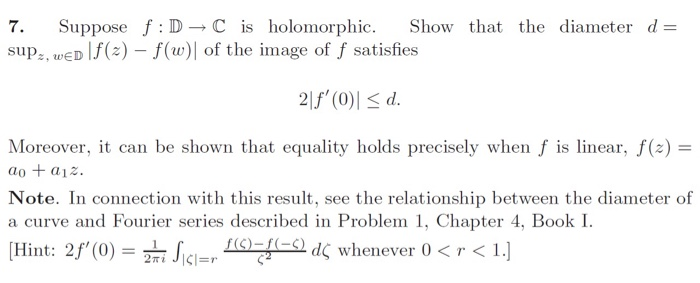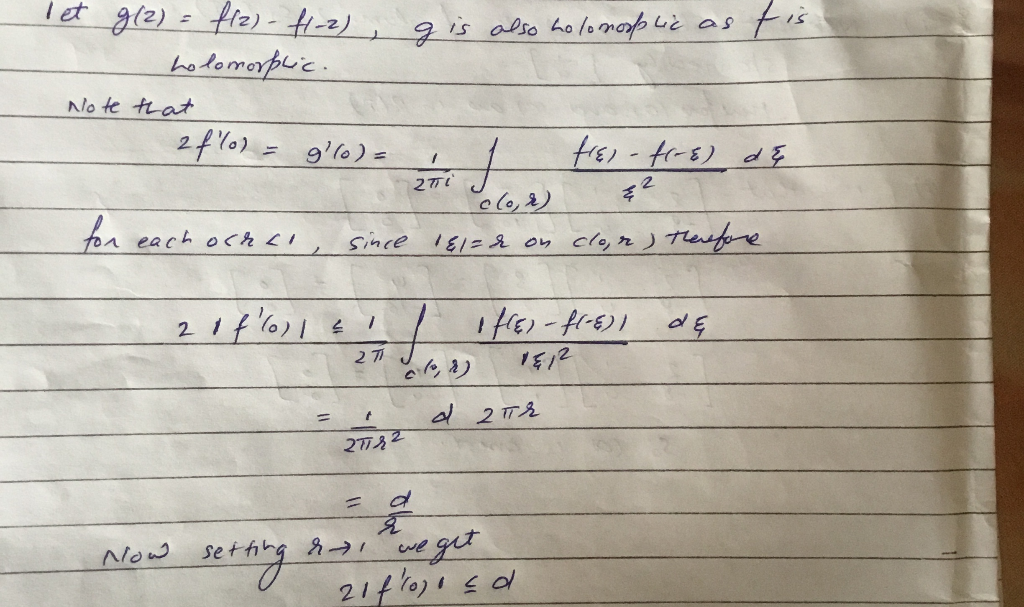Homework Help Question & Answers

# 7. Suppose f: D→C is holomorphic. Show that the diameter d= sup,, wED f(a)-f(w)of the image of f ...7. Suppose f: D→C is holomorphic. Show that the diameter d= sup,, wED f(a)-f(w)of the image of f satisfies Moreover, it can be shown that equality holds precisely when f is linear, f(z)- Note. In connection with this result, see the relationship between the diameter of a curve and Fourier seri . es described in Problem 1, Chapter 4, Book I -, Layer d' whenever 0

#### Homework Answers

ReportAnswer #1Add Homework Help Answer
##### Add Answer of: 7. Suppose f: D→C is holomorphic. Show that the diameter d= sup,, wED f(a)-f(w)of the image of f ...
Your Answer: Your Name: What's your source?
Not the answer you're looking for? Ask your own homework help question. Our experts will answer your question WITHIN MINUTES for Free.
More Homework Help Questions Additional questions in this topic.

• #### Find sin(x/2) if sin(x)= -0.4 and 3pi/2 < or equal to (x) < or equal to 2pi Let's use cos 2A = 1 - 2sin<sup>2</sup> A and we can match cos x = 1 - 2sin<sup>2</sup> (x/2) so we will need cos x we know sin x = -

Need Online Homework Help?

Get FREE EXPERT Answers
WITHIN MINUTES
Related Questions